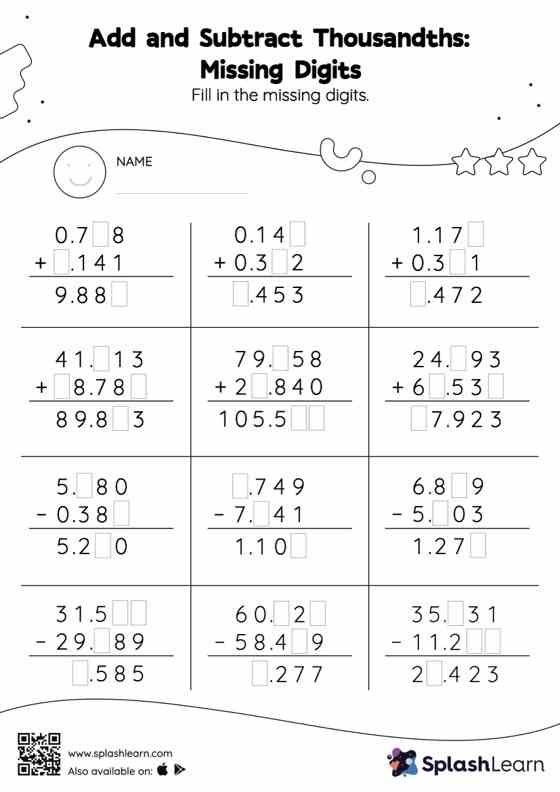# Add and Subtract Thousandths: Missing Digits Worksheet

Home > Add and Subtract Thousandths: Missing DigitsThis add and subtract thousandths worksheet has a set of problems curated to ensure that your child becomes more proficient in addition and subtraction. In this worksheet, students add and subtract decimals by aligning the decimal points and using zero as a placeholder. They then use the relationship between addition and subtraction to find the missing number in the add and subtract thousandths worksheet. This worksheet is about practicing the column method in which numbers are written one on top of another. In this method, students use their place value understanding to solve the problems.